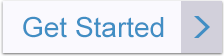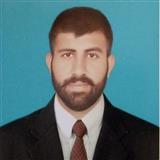Pure_Mentor1122Away
Tutor in:
Calculus
Languages:
English

I can provide expert guidance in Algebra, Applied Mathematics, Calculus, Geometry, Linear Algebra, Number Theory, Set Theory, Probability, Statistics and Mechanics.

Degrees

Ph.D. in Mathematical Sciencies M.S. in Statistics M.Sc. in Applied Mathematics Bachelor Degree in Mathematics and Physics

My Tutoring Service

I am a Mathematics Professor with 15 years of instructing knowledge. I can show subjects identified with Algebra, Calculus, Trigonometry, Geometry, Number Theory, Linear Algebra, Statistics, Probability and so on. I stressed more on critical thinking abilities so you can comprehend the ideas identified with Probability.

Experience & Qualifications

I have more than 15 Years of Tutoring background in different fields of Mathematics like Algebra, Calculus, Geometry, Trigonometry and Statistics. I have created aptitude to take care of math issues utilizing Statistical Software.